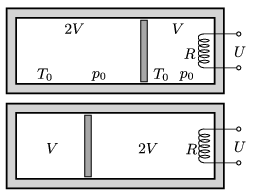Mathematical and Physical Journal
for High Schools
Issued by the MATFUND Foundation
 Already signed up? New to KöMaL?

#Problem P. 4825. (March 2016)

P. 4825. A thermally insulated container is divided into two parts of volumes $\displaystyle V$ and $\displaystyle 2V$ by a thermally insulated piston. Initially both parts contain air at the temperature of $\displaystyle T_0=300~\rm K$, and at the pressure of $\displaystyle p_0=10^5~\rm Pa$. An electric heater of resistance $\displaystyle R=100\,\Omega$, rated at $\displaystyle U=230$ V, is built into that part which has a volume of $\displaystyle V=2$ litres. The heater is operated until the values of the volume of the gas at the two parts swap over'', that is, in accordance with the figure the volume of the gas at the left hand-side of the container becomes $\displaystyle V$, and the volume of the gas at the right hand-side becomes $\displaystyle 2V$.$\displaystyle a)$ What are the temperature values at the two parts of the container at the end?

$\displaystyle b)$ How long did the process take?

(5 pont)

Deadline expired on April 11, 2016.

### Statistics:

 59 students sent a solution. 5 points: Asztalos Bogdán, Balogh Menyhért, Bartók Imre, Bekes Nándor, Blum Balázs, Csorba Benjámin, Csuha Boglárka, Di Giovanni András, Édes Lili, Elek Péter, Fajszi Bulcsú, Fehér 169 Szilveszter, Fekete Balázs Attila, Forrai Botond, Iván Balázs, Jakus Balázs István, Kasza Bence, Kormányos Hanna Rebeka, Kovács Péter Tamás, Körmöczi Dávid, Krasznai Anna, Mány Bence, Marozsák Tóbiás , Molnár Mátyás, Németh 777 Róbert, Németh Flóra Boróka, Olosz Adél, Páhoki Tamás, Pataki 245 Attila, Pázmán Előd, Pszota Máté, Sal Kristóf, Sallai Krisztina, Szentivánszki Soma , Tófalusi Ádám, Tomcsányi Gergely, Varga-Umbrich Eszter, Wiandt Péter, Zöllner András. 4 points: Farkas Domonkos, Gémes Antal, Ghada Alshalan, Hornák Bence, Szántó Benedek, Tibay Álmos. 3 points: 5 students. 1 point: 3 students. 0 point: 6 students.

Problems in Physics of KöMaL, March 2016